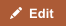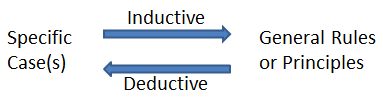Deductive reasoning uses given information, premises or accepted general rules to reach a proven conclusion. On the other hand, inductive logic or reasoning involves making generalizations based upon behavior observed in specific cases. Deductive arguments are either valid or invalid. But inductive logic allows for the conclusions to be wrong even if the premises upon which it is based are correct. So inductive arguments are either strong or weak.

## Comparison chart

Deductive versus Inductive comparison chartDeductiveInductive
Introduction (from Wikipedia) Deductive reasoning, also called deductive logic, is the process of reasoning from one or more general statements regarding what is known to reach a logically certain conclusion. Inductive reasoning, also called induction or bottom-up logic, constructs or evaluates general propositions that are derived from specific examples.
Arguments Arguments in deductive logic are either valid or invalid. Invalid arguments are always unsound. Valid arguments are sound only if the premises they are based upon are true. Arguments in inductive reasoning are either strong or weak. Weak arguments are always uncogent. Strong arguments are cogent only if the premises they are based upon are true.
Validity of conclusions Conclusions can be proven to be valid if the premises are known to be true. Conclusions may be incorrect even if the argument is strong and the premises are true.Deductive reasoning applies general rules to make conclusions about specific cases. Inductive reasoning observes patterns in specific cases to infer conclusions about general rules.

For example: All men are mortal. John is a man. Therefore John is mortal. This is an example of valid deductive reasoning. On the other hand, here's an example of inductive reasoning: Most men are right-handed. John is a man. Therefore, John must be right-handed. The strength of this inductive argument depends upon the percentage of left-handed people in the population. In any case, the conclusion may well end up being invalid because inductive reasoning does not guarantee validity of the conclusions.

## What is Deductive Reasoning?

Deductive reasoning (top-down logic) contrasts with inductive reasoning (bottom-up logic), and generally starts with one or more general statements or premises to reach a logical conclusion. If the premises are true, the conclusion must be valid. Deductive resasoning is used by scientists and mathematicians to prove their hypotheses.

### Sound or Unsound arguments

With deductive reasoning, arguments may be valid or invalid, sound or unsound. If the logic is correct, i.e. the conclusion flows from the premises, then the arguments are valid. However, valid arguments may be sound or unsound. If the premises used in the valid argument are true, then the argument is sound otherwise it is unsound.

For example,

1. All men have ten fingers.
2. John is a man.
3. Therefore, John has ten fingers.

This argument is logical and valid. However, the premise "All men have ten fingers." is incorrect because some people are born with 11 fingers. Therefore, this is an unsound argument. Note that all invalid arguments are also unsound.

### Types of deductive logic

#### Law of detachment

A single conditional statement is made, and a hypothesis (P) is stated. The conclusion (Q) is then deduced from the statement and the hypothesis. For example, using the law of detachment in the form of an if-then statement: (1.) If an angle A>90°, then A is an obtuse angle. (2.) A=125°. (3.) Therefore, A is an obtuse angle.

#### The law of Syllogism

The law of syllogism takes two conditional statements and forms a conclusion by combining the hypothesis of one statement with the conclusion of another. For example, (1.) If the brakes fail, the car will not stop. (2.) If the car does not stop, there will be an accident. (3.) Therefore, If the brakes fail, there will be an accident.

We deduced the final statement by combining the hypothesis of the first statement with the conclusion of the second statement.

## What is Inductive Reasoning?

Inductive reasoning, or induction, is reasoning from a specific case or cases and deriving a general rule. This is against the scientific method. It makes generalizations by observing patterns and drawing inferences that may well be incorrect.

### Cogent and Uncogent Arguments

Strong arguments are ones where if the premise is true then the conclusion is very likely to be true. Conversely, weak inductive arguments are such that they may be false even if the premises they are based upon are true.

If the argument is strong and the premises it is based upon are true, then it is said to be a cogent argument. If the argument is weak or the premises it flows from are false or unproven, then the argument is said to be uncogent.

For example, here is an example of a strong argument.

1. There are 20 cups of ice cream in the freezer.
2. 18 of them are vanilla flavored.
3. Therefore, all cups of ice cream are vanilla.

If in the previous argument premise #2 was that 2 of the cups are vanilla, then the conclusion that all cups are vanilla would be based upon a weak argument. In either case, all premises are true and the conclusion may be incorrect, but the strength of the argument varies.

### Types of Inductive Reasoning

#### Generalization

A generalization proceeds from a premise about a sample to a conclusion about the population. For example, (1.) A sample S from population P is chose. Q percentage of the sample S has attribute A. (2.) Therefore, Q percentage of the population P has attribute A.

#### Statistical Syllogisms

A statistical syllogism proceeds from a generalization to a conclusion about an individual. For example, (1.) A proportion Q of population P has attribute A. (2.) An individual X is a member of P. (3.) Therefore, there is a probability which corresponds to Q that X has an attribute A.

## More Examples

### Examples of Deductive Reasoning

Quadrilateral ABCD has sides AB ll CD (parallel) and sides BC ll AD. Prove that it is a parallelogram. In order to prove this, we have to use the general statements given about the quadrilateral and reach a logical conclusion.

Another example of deductive logic is the following reasoning:

1. All labrador retrievers are dogs.
2. Some labrador retrievers are pets.
3. Therefore, some dogs are pets.

### Examples of Inductive Reasoning

If the three consecutive shapes are triangle, square and pentagon which would be the next shape? If the reasoner observes the pattern, she will observe that the number of sides in the shape increase by one and so a generalization of this pattern would lead her to conclude that the next shape in the sequence would be a hexagon.

## Applications of Inductive and Deductive Reasoning

• Deduction can also be temporarily used to test an induction by applying it elsewhere.
• A good scientific law is highly generalized like that in Inductive reasoning and may be applied in many situations to explain other phenomena.
• Deductive reasoning is used to deduce many experiments and prove a general rule.

## Bias

Inductive reasoning is also known as hypothesis construction because any conclusions made are based on current knowledge and predictions. As with deductive arguments, biases can distort the proper application of inductive argument, which prevents the reasoner from forming the most logical conclusion based on the clues.

### Availability Heuristic

The availability heuristic causes the reasoner to depend primarily upon information that is readily available. People have a tendency to rely on information that is easily accessible in the world around them. This can introduce bias in inductive reasoning.

### Confirmation bias

The confirmation bias is based on the natural tendency to confirm, rather than to deny a current hypothesis. For example, for several centuries it was believed that the sun and planets orbit the earth.